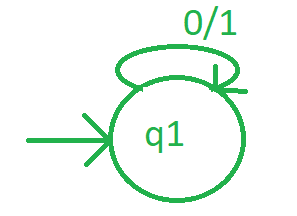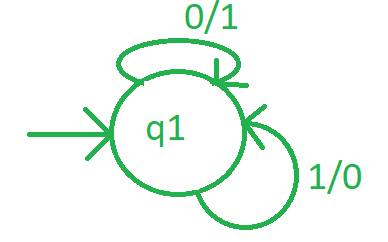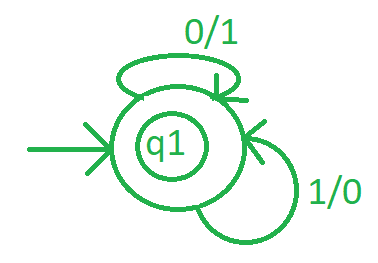# Mealy Machine for 1’s Complement

• Last Updated : 07 Jul, 2021

After converting a number to its binary form, replace every one of the number with 0 and every 0 with 1, then the resulting number will be known as 1, s complement of that binary number.

```Input-1 :
101010
Output-1 :
010101

Input-2 :
1110100
Output-2 :
0001011 ```

Approach :

1. First make a initial state.
2. Then convert each 0 to 1 and each 1 to 0, and reach to next possible state.
3. after performing step 2 on each digit of binary number, reach at some final state to complete the process.

Design Mealy Machine :

Step-1:
Take a initial state say q1, and if the input is found 0, convert it to 1, remain at same state and check for another input alphabet.Step-2:
If state q1 takes input alphabet is 1, then convert it to 0 and remain at same state.Step-3:As after converting all alphabet, it is on same state then this state will be final state as well.Example :
Suppose a string 10001 and start parsing from left to right. Every 0 will be replaced by 1 and every one is replaces by 0. Then the output is 01110.

My Personal Notes arrow_drop_up